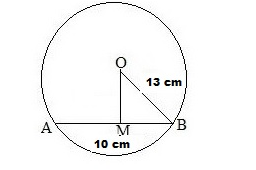# The radius of a circle is 13 cm and the length of one of its chords is 10 cm.

Question:

The radius of a circle is 13 cm and the length of one of its chords is 10 cm. The distance of the chord from the centre is

(a) 11.5 cm
(b) 12 cm

(c) $\sqrt{69} \mathrm{~cm}$

(d) 23 cm

Solution:

(b) 12 cm
Let AB be the chord of the given circle with centre O and a radius of 13 cm.
Then, AB = 10 cm and OB = 13 cmFrom O, draw OM perpendicular to AB.
We know that the perpendicular from the centre of a circle to a chord bisects the chord.

$\therefore B M=\left(\frac{10}{2}\right) \mathrm{cm}=5 \mathrm{~cm}$

From the right ΔOMB, we have:

$\mathrm{OB}^{2}=\mathrm{OM}^{2}+\mathrm{MB}^{2}$

$\Rightarrow 13^{2}=\mathrm{OM}^{2}+5^{2}$

$\Rightarrow 169=\mathrm{OM}^{2}+25$

$\Rightarrow \mathrm{OM}^{2}=(169-25)=144$

$\Rightarrow \mathrm{OM}=\sqrt{144} \mathrm{~cm}=12 \mathrm{~cm}$

Hence, the distance of the chord from the centre is 12 cm.